## C# || How To Find The Nearest Exit From Entrance In Maze Using C#The following is a module with functions which demonstrates how to find the nearest exit from the entrance in a maze using C#.

1. Nearest Exit – Problem Statement

You are given an m x n matrix maze (0-indexed) with empty cells (represented as ‘.’) and walls (represented as ‘+’). You are also given the entrance of the maze, where entrance = [entrancerow, entrancecol] denotes the row and column of the cell you are initially standing at.

In one step, you can move one cell up, down, left, or right. You cannot step into a cell with a wall, and you cannot step outside the maze. Your goal is to find the nearest exit from the entrance. An exit is defined as an empty cell that is at the border of the maze. The entrance does not count as an exit.

Return the number of steps in the shortest path from the entrance to the nearest exit, or -1 if no such path exists.

Example 1: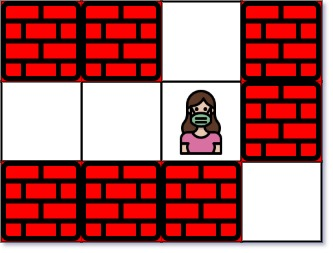``` Input: maze = [["+","+",".","+"],[".",".",".","+"],["+","+","+","."]], entrance = [1,2] Output: 1 Explanation: There are 3 exits in this maze at [1,0], [0,2], and [2,3]. Initially, you are at the entrance cell [1,2]. - You can reach [1,0] by moving 2 steps left. - You can reach [0,2] by moving 1 step up. It is impossible to reach [2,3] from the entrance. Thus, the nearest exit is [0,2], which is 1 step away. ```

Example 2:``` Input: maze = [["+","+","+"],[".",".","."],["+","+","+"]], entrance = [1,0] Output: 2 Explanation: There is 1 exit in this maze at [1,2]. [1,0] does not count as an exit since it is the entrance cell. Initially, you are at the entrance cell [1,0]. - You can reach [1,2] by moving 2 steps right. Thus, the nearest exit is [1,2], which is 2 steps away. ```

Example 3: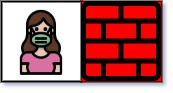``` Input: maze = [[".","+"]], entrance = [0,0] Output: -1 Explanation: There are no exits in this maze. ```

2. Nearest Exit – Solution

The following is a solution which demonstrates how find the nearest exit from the entrance in a maze.

``` 2. Nearest Exit - Solution C# // ============================================================================ // Author: Kenneth Perkins // Date: Dec 1, 2022 // Taken From: http://programmingnotes.org/ // File: Solution.cs // Description: Demonstrates how to find nearest exit from entrance in maze // ============================================================================ public class Solution { public int NearestExit(char[][] maze, int[] entrance) { int rows = maze.Length; int cols = maze.Length; var directions = new List<KeyValuePair<int, int>>() { // Direction coordinates: (y, x) new KeyValuePair<int, int>(1, 0), new KeyValuePair<int, int>(-1, 0), new KeyValuePair<int, int>(0, 1), new KeyValuePair<int, int>(0, -1) }; char VISITED = '+'; char NOT_VISITED = '.'; // Mark the entrance as visited since its not a exit. int startRow = entrance; int startCol = entrance; maze[startRow][startCol] = VISITED; // Start BFS from the entrance, and use a queue `queue` to store all // the cells to be visited. var queue = new Queue<int[]>(); queue.Enqueue(new int[]{startRow, startCol, 0}); while (queue.Count > 0) { int[] currState = queue.Dequeue(); int currRow = currState; int currCol = currState; int currDistance = currState; // For the current cell, check its four neighbor cells. foreach (var dir in directions) { int nextRow = currRow + dir.Key; int nextCol = currCol + dir.Value; // If there exists an unvisited empty neighbor: if (IsValidCell(maze, nextRow, nextCol) && maze[nextRow][nextCol] == NOT_VISITED) { // If this empty cell is an exit, return distance + 1. if (nextRow == 0 || nextRow == rows - 1 || nextCol == 0 || nextCol == cols - 1) { return currDistance + 1; } // Otherwise, add this cell to 'queue' and mark it as visited. maze[nextRow][nextCol] = VISITED; queue.Enqueue(new int[]{nextRow, nextCol, currDistance + 1}); } } } // If we finish iterating without finding an exit, return -1. return -1; } private bool IsValidCell(char[][] grid, int row, int col) { return row >= 0 && row < grid.Length && col >= 0 && col < grid[row].Length; } }// http://programmingnotes.org/ 12345678910111213141516171819202122232425262728293031323334353637383940414243444546474849505152535455565758596061626364 // ============================================================================//    Author: Kenneth Perkins//    Date:   Dec 1, 2022//    Taken From: http://programmingnotes.org///    File:  Solution.cs//    Description: Demonstrates how to find nearest exit from entrance in maze// ============================================================================public class Solution {    public int NearestExit(char[][] maze, int[] entrance) {        int rows = maze.Length;        int cols = maze.Length;        var directions = new List<KeyValuePair<int, int>>() {            // Direction coordinates: (y, x)            new KeyValuePair<int, int>(1, 0),            new KeyValuePair<int, int>(-1, 0),            new KeyValuePair<int, int>(0, 1),            new KeyValuePair<int, int>(0, -1)        };        char VISITED = '+';        char NOT_VISITED = '.';         // Mark the entrance as visited since its not a exit.        int startRow = entrance;        int startCol = entrance;        maze[startRow][startCol] = VISITED;         // Start BFS from the entrance, and use a queue `queue` to store all        // the cells to be visited.        var queue = new Queue<int[]>();        queue.Enqueue(new int[]{startRow, startCol, 0});         while (queue.Count > 0) {            int[] currState = queue.Dequeue();            int currRow = currState;            int currCol = currState;            int currDistance = currState;             // For the current cell, check its four neighbor cells.            foreach (var dir in directions) {                int nextRow = currRow + dir.Key;                int nextCol = currCol + dir.Value;                 // If there exists an unvisited empty neighbor:                if (IsValidCell(maze, nextRow, nextCol) && maze[nextRow][nextCol] == NOT_VISITED) {                    // If this empty cell is an exit, return distance + 1.                    if (nextRow == 0 || nextRow == rows - 1 || nextCol == 0 || nextCol == cols - 1) {                        return currDistance + 1;                    }                     // Otherwise, add this cell to 'queue' and mark it as visited.                    maze[nextRow][nextCol] = VISITED;                    queue.Enqueue(new int[]{nextRow, nextCol, currDistance + 1});                }            }        }         // If we finish iterating without finding an exit, return -1.        return -1;    }     private bool IsValidCell(char[][] grid, int row, int col) {        return row >= 0 && row < grid.Length && col >= 0 && col < grid[row].Length;    }}// http://programmingnotes.org/ ```

QUICK NOTES:
The highlighted lines are sections of interest to look out for.

The code is heavily commented, so no further insight is necessary. If you have any questions, feel free to leave a comment below.

Once compiled, you should get this as your output for the example cases:

``` 1 2 -1 ```

## C# || Two Sum IV – How To Get Two Numbers In Binary Search Tree Equal To Target Value Using C#The following is a module with functions which demonstrates how to get two numbers in a binary search tree equal to target value using C#.

1. Find Target – Problem Statement

Given the root of a Binary Search Tree and a target number k, return true if there exist two elements in the BST such that their sum is equal to the given target.

Example 1:``` Input: root = [5,3,6,2,4,null,7], k = 9 Output: true ```

Example 2: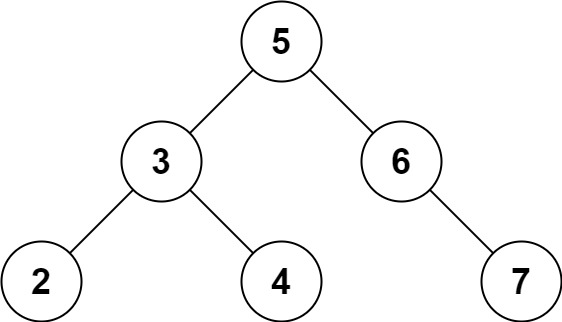``` Input: root = [5,3,6,2,4,null,7], k = 28 Output: false ```

2. Find Target – Solution

The following are two solutions which demonstrates how to get two numbers in a binary search tree equal to target value.

Both solutions use a set to keep track of the items already seen.

Each time a new node is encountered, we subtract the target value from the current node value. If the difference amount from subtracting the two numbers exists in the set, a 2 sum combination exists in the tree

1. Recursive

The following solution uses Depth First Search when looking for the target value.

``` 2. Find Target - Solution - Recursive C# // ============================================================================ // Author: Kenneth Perkins // Date: Oct 9, 2022 // Taken From: http://programmingnotes.org/ // File: Solution.cs // Description: Demonstrates how to get two numbers equal to target value // ============================================================================ public class Solution { public bool FindTarget(TreeNode root, int k) { return Traverse(root, k, new HashSet<int>()); } private bool Traverse(TreeNode node, int k, HashSet<int> seen) { if (node == null) { return false; } // Get remaining value var remaining = k - node.val; // Check if remaining value has been seen if (seen.Contains(remaining)) { return true; } // Add current node value to items seen seen.Add(node.val); // Keep traversing left and right looking for 2 sum return Traverse(node.left, k, seen) || Traverse(node.right, k, seen); } }// http://programmingnotes.org/ 123456789101112131415161718192021222324252627282930 // ============================================================================//    Author: Kenneth Perkins//    Date:   Oct 9, 2022//    Taken From: http://programmingnotes.org///    File:  Solution.cs//    Description: Demonstrates how to get two numbers equal to target value// ============================================================================public class Solution {    public bool FindTarget(TreeNode root, int k) {        return Traverse(root, k, new HashSet<int>());    }     private bool Traverse(TreeNode node, int k, HashSet<int> seen) {        if (node == null) {            return false;        }        // Get remaining value        var remaining = k - node.val;         // Check if remaining value has been seen        if (seen.Contains(remaining)) {            return true;        }        // Add current node value to items seen        seen.Add(node.val);         // Keep traversing left and right looking for 2 sum        return Traverse(node.left, k, seen) || Traverse(node.right, k, seen);    }}// http://programmingnotes.org/ ```

2. Iterative

The following solution uses Breadth First Search when looking for the target value.

``` 2. Find Target - Solution - Iterative C# // ============================================================================ // Author: Kenneth Perkins // Date: Oct 9, 2022 // Taken From: http://programmingnotes.org/ // File: Solution.cs // Description: Demonstrates how to get two numbers equal to target value // ============================================================================ public class Solution { public bool FindTarget(TreeNode root, int k) { if (root == null) { return false; } // Declare stack var stack = new Stack<TreeNode>(); // Keep track of values seen var seen = new HashSet<int>(); // Add root to stack stack.Push(root); // Loop through items on the stack while (stack.Count > 0) { // Get current node var node = stack.Pop(); // Get remaining value var remaining = k - node.val; // Check if remaining value has been seen if (seen.Contains(remaining)) { return true; } else { // Keep traversing left and right looking for 2 sum if (node.left != null) { stack.Push(node.left); } if (node.right != null) { stack.Push(node.right); } } // Add current node value to items seen seen.Add(node.val); } return false; } }// http://programmingnotes.org/ 1234567891011121314151617181920212223242526272829303132333435363738394041424344454647 // ============================================================================//    Author: Kenneth Perkins//    Date:   Oct 9, 2022//    Taken From: http://programmingnotes.org///    File:  Solution.cs//    Description: Demonstrates how to get two numbers equal to target value// ============================================================================public class Solution {    public bool FindTarget(TreeNode root, int k) {        if (root == null) {            return false;        }        // Declare stack        var stack = new Stack<TreeNode>();         // Keep track of values seen        var seen = new HashSet<int>();         // Add root to stack        stack.Push(root);         // Loop through items on the stack        while (stack.Count > 0) {            // Get current node            var node = stack.Pop();             // Get remaining value            var remaining = k - node.val;             // Check if remaining value has been seen            if (seen.Contains(remaining)) {                return true;            } else {                // Keep traversing left and right looking for 2 sum                if (node.left != null) {                    stack.Push(node.left);                }                if (node.right != null) {                    stack.Push(node.right);                }            }            // Add current node value to items seen            seen.Add(node.val);        }        return false;    }}// http://programmingnotes.org/ ```

QUICK NOTES:
The highlighted lines are sections of interest to look out for.

The code is heavily commented, so no further insight is necessary. If you have any questions, feel free to leave a comment below.

Once compiled, you should get this as your output for the example cases:

``` true false ```

## C# || How To Find The Shortest Clear Path In A Binary Matrix Using C#The following is a module with functions which demonstrates how to find the shortest clear path in a binary matrix using C#.

1. Shortest Path Binary Matrix – Problem Statement

Given an n x n binary matrix grid, return the length of the shortest clear path in the matrix. If there is no clear path, return -1.

A clear path in a binary matrix is a path from the top-left cell (i.e., (0, 0)) to the bottom-right cell (i.e., (n – 1, n – 1)) such that:

• All the visited cells of the path are 0.
• All the adjacent cells of the path are 8-directionally connected (i.e., they are different and they share an edge or a corner).

The length of a clear path is the number of visited cells of this path.

Example 1: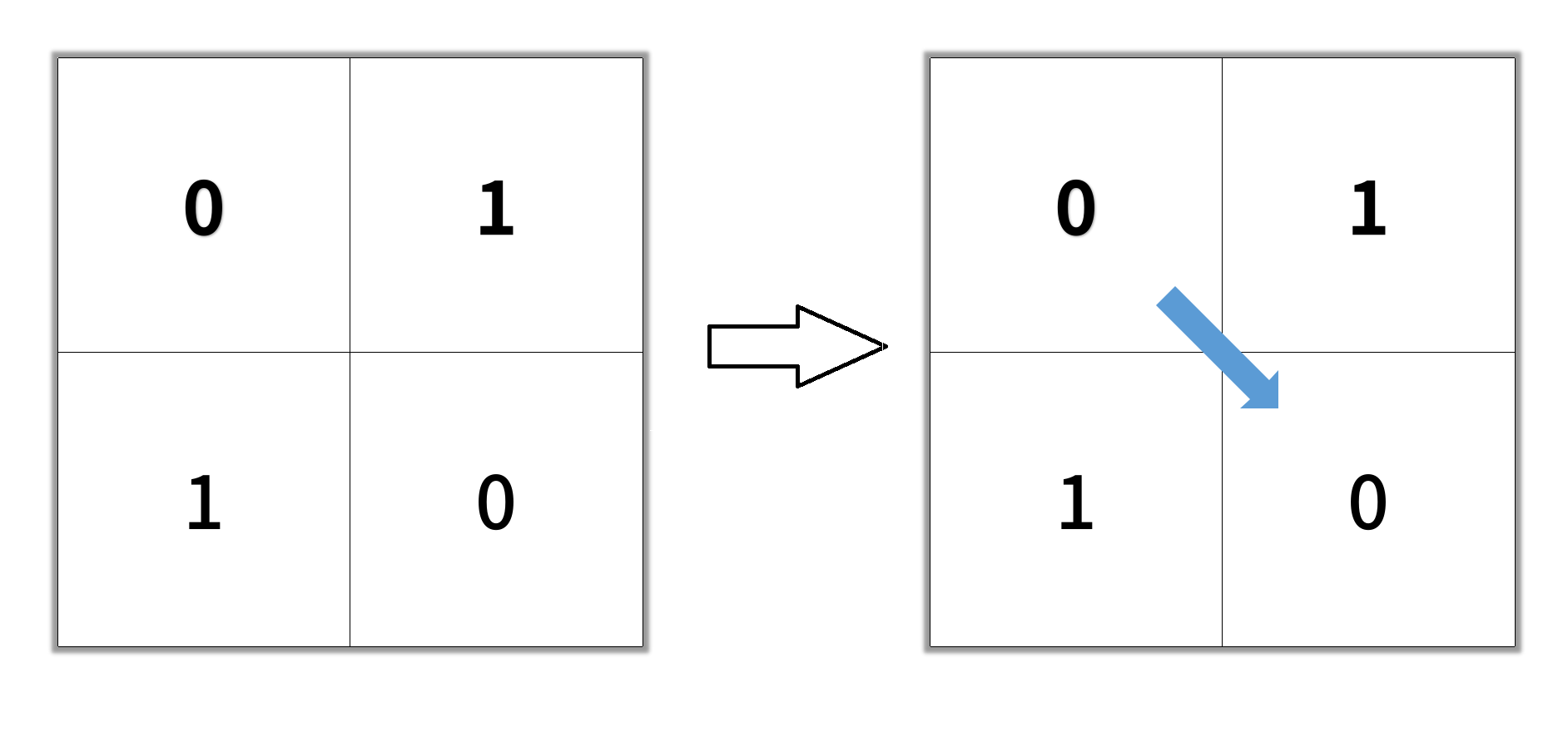``` Input: grid = [[0,1],[1,0]] Output: 2 ```

Example 2: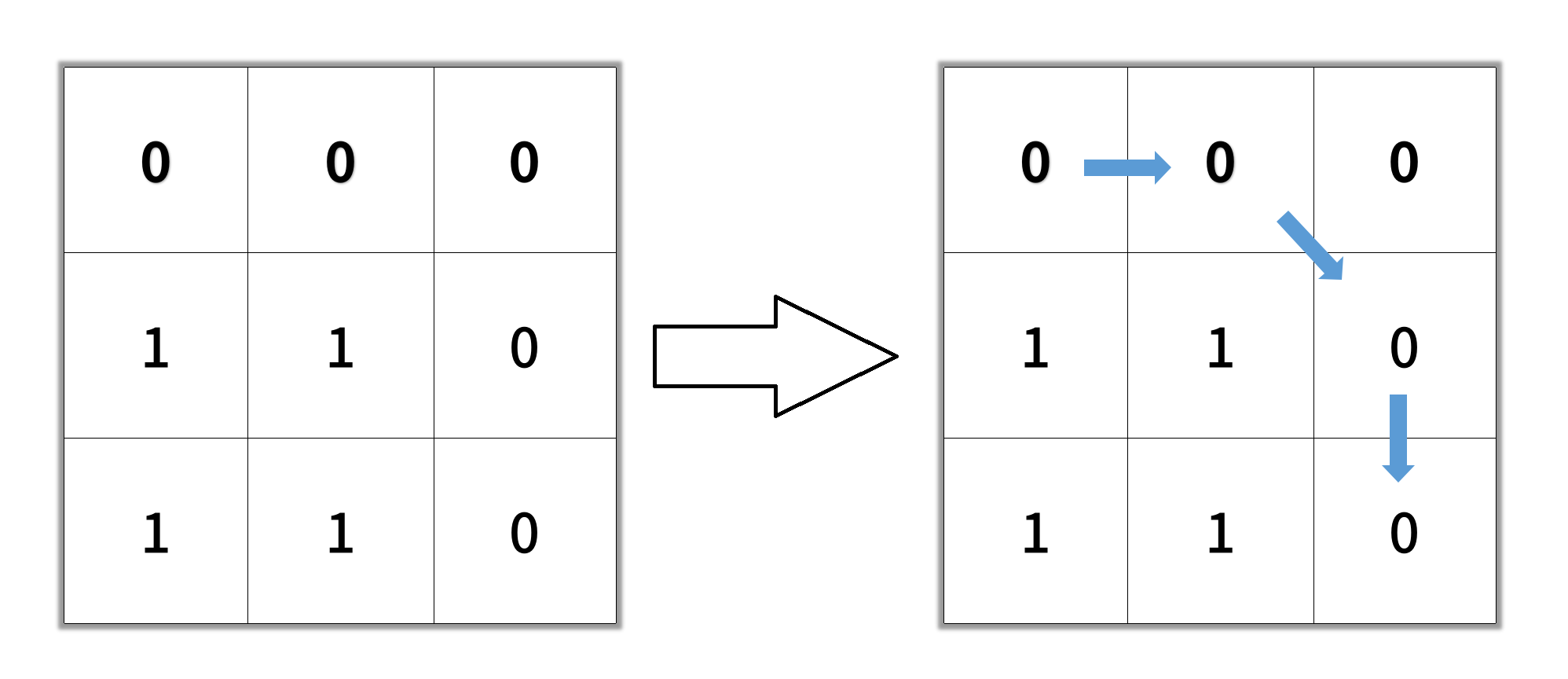``` Input: grid = [[0,0,0],[1,1,0],[1,1,0]] Output: 4 ```

Example 3:

``` Input: grid = [[1,0,0],[1,1,0],[1,1,0]] Output: -1 ```

2. Shortest Path Binary Matrix – Solution

The following is a solution which demonstrates how find the shortest clear path in a binary matrix.

``` 2. Shortest Path Binary Matrix - Solution C# // ============================================================================ // Author: Kenneth Perkins // Date: Jun 3, 2022 // Taken From: http://programmingnotes.org/ // File: Solution.cs // Description: Demonstrates how to find shortest path binary matrix // ============================================================================ public class Solution { // Search directions List<KeyValuePair<int, int>> directions = new List<KeyValuePair<int, int>>() { // Direction coordinates: (y, x) new KeyValuePair<int, int>(0, -1), new KeyValuePair<int, int>(0, 1), new KeyValuePair<int, int>(-1, 0), new KeyValuePair<int, int>(1, 0), new KeyValuePair<int, int>(1, 1), new KeyValuePair<int, int>(-1, -1), new KeyValuePair<int, int>(-1, 1), new KeyValuePair<int, int>(1, -1) }; public int ShortestPathBinaryMatrix(int[][] grid) { var NOT_VISITED = 0; var VISITED = 1; if (grid == VISITED) { return -1; } var queue = new Queue<KeyValuePair<int, int>>(); queue.Enqueue(new KeyValuePair<int, int>(0, 0)); grid = VISITED; var result = 0; while(queue.Count > 0) { ++result; for (var itemCount = queue.Count; itemCount > 0; --itemCount) { var current = queue.Dequeue(); // Get the current coordinates var row = current.Key; var col = current.Value; if (row == grid.Length - 1 && col == grid[row].Length - 1) { return result; } // Search in different directions foreach (var direction in directions) { var newRow = row + direction.Key; var newCol = col + direction.Value; if (IsValidCell(grid, newRow, newCol) && grid[newRow][newCol] == NOT_VISITED) { grid[newRow][newCol] = VISITED; queue.Enqueue(new KeyValuePair<int, int>(newRow, newCol)); } } } } return -1; } private bool IsValidCell(int[][] grid, int row, int col) { return row >= 0 && row < grid.Length && col >= 0 && col < grid[row].Length; } }// http://programmingnotes.org/ 123456789101112131415161718192021222324252627282930313233343536373839404142434445464748495051525354555657585960616263646566 // ============================================================================//    Author: Kenneth Perkins//    Date:   Jun 3, 2022//    Taken From: http://programmingnotes.org///    File:  Solution.cs//    Description: Demonstrates how to find shortest path binary matrix// ============================================================================public class Solution {    // Search directions    List<KeyValuePair<int, int>> directions = new List<KeyValuePair<int, int>>() {        // Direction coordinates: (y, x)        new KeyValuePair<int, int>(0, -1),        new KeyValuePair<int, int>(0, 1),        new KeyValuePair<int, int>(-1, 0),        new KeyValuePair<int, int>(1, 0),        new KeyValuePair<int, int>(1, 1),        new KeyValuePair<int, int>(-1, -1),        new KeyValuePair<int, int>(-1, 1),        new KeyValuePair<int, int>(1, -1)    };     public int ShortestPathBinaryMatrix(int[][] grid) {        var NOT_VISITED = 0;        var VISITED = 1;         if (grid == VISITED) {            return -1;        }         var queue = new Queue<KeyValuePair<int, int>>();        queue.Enqueue(new KeyValuePair<int, int>(0, 0));        grid = VISITED;         var result = 0;        while(queue.Count > 0) {            ++result;            for (var itemCount = queue.Count; itemCount > 0; --itemCount) {                var current = queue.Dequeue();                 // Get the current coordinates                var row = current.Key;                var col = current.Value;                 if (row == grid.Length - 1 && col == grid[row].Length - 1) {                    return result;                }                 // Search in different directions                foreach (var direction in directions) {                    var newRow = row + direction.Key;                    var newCol = col + direction.Value;                     if (IsValidCell(grid, newRow, newCol) && grid[newRow][newCol] == NOT_VISITED) {                        grid[newRow][newCol] = VISITED;                        queue.Enqueue(new KeyValuePair<int, int>(newRow, newCol));                    }                }            }        }        return -1;    }     private bool IsValidCell(int[][] grid, int row, int col) {        return row >= 0 && row < grid.Length && col >= 0 && col < grid[row].Length;    }}// http://programmingnotes.org/ ```

QUICK NOTES:
The highlighted lines are sections of interest to look out for.

The code is heavily commented, so no further insight is necessary. If you have any questions, feel free to leave a comment below.

Once compiled, you should get this as your output for the example cases:

``` 2 4 -1 ```

## C# || Jump Game III – How To Check If You Can Reach Target Value In Array Using C#The following is a module with functions which demonstrates how to check if you can reach a target value in an array using C#.

1. Can Reach – Problem Statement

Given an array of non-negative integers arr, you are initially positioned at start index of the array. When you are at index i, you can jump to i + arr[i] or i – arr[i], check if you can reach to any index with value 0.

Notice that you can not jump outside of the array at any time.

Example 1:

``` Input: arr = [4,2,3,0,3,1,2], start = 5 Output: true Explanation: All possible ways to reach at index 3 with value 0 are: index 5 -> index 4 -> index 1 -> index 3 index 5 -> index 6 -> index 4 -> index 1 -> index 3 ```

Example 2:

``` Input: arr = [4,2,3,0,3,1,2], start = 0 Output: true Explanation: One possible way to reach at index 3 with value 0 is: index 0 -> index 4 -> index 1 -> index 3 ```

Example 3:

``` Input: arr = [3,0,2,1,2], start = 2 Output: false Explanation: There is no way to reach at index 1 with value 0. ```

2. Can Reach – Solution

The following are two solutions which demonstrates how to check if you can reach a target value in an array.

The following solution uses Depth First Search when looking for the target value.

``` 2. Can Reach - DFS - Solution C# // ============================================================================ // Author: Kenneth Perkins // Date: Dec 8, 2021 // Taken From: http://programmingnotes.org/ // File: Solution.cs // Description: Determines if a target value can be reached in an array // ============================================================================ public class Solution { public bool CanReach(int[] arr, int start) { var visited = new bool[arr.Length]; return DFS(arr, start, visited); } public bool DFS(int[] arr, int currentIndex, bool[] visited) { // Make sure parameters are in bounds if (currentIndex < 0 || currentIndex >= arr.Length) { return false; } // Check to see if the index has been visited if (visited[currentIndex]) { return false; } // Check to see if we found the target value if (arr[currentIndex] == 0) { return true; } // Mark current index as visited visited[currentIndex] = true; // Keep searching forwards and backwards var forwards = currentIndex + arr[currentIndex]; var backwards = currentIndex - arr[currentIndex]; return DFS(arr, backwards, visited) || DFS(arr, forwards, visited); } }// http://programmingnotes.org/ 1234567891011121314151617181920212223242526272829303132333435 // ============================================================================//    Author: Kenneth Perkins//    Date:   Dec 8, 2021//    Taken From: http://programmingnotes.org///    File:  Solution.cs//    Description: Determines if a target value can be reached in an array// ============================================================================public class Solution {    public bool CanReach(int[] arr, int start) {        var visited = new bool[arr.Length];        return DFS(arr, start, visited);    }    public bool DFS(int[] arr, int currentIndex, bool[] visited) {        // Make sure parameters are in bounds        if (currentIndex < 0 || currentIndex >= arr.Length) {            return false;        }        // Check to see if the index has been visited        if (visited[currentIndex]) {            return false;        }        // Check to see if we found the target value        if (arr[currentIndex] == 0) {            return true;        }        // Mark current index as visited        visited[currentIndex] = true;         // Keep searching forwards and backwards        var forwards = currentIndex + arr[currentIndex];        var backwards = currentIndex - arr[currentIndex];         return DFS(arr, backwards, visited) || DFS(arr, forwards, visited);    }}// http://programmingnotes.org/ ```

The following solution uses Breadth First Search when looking for the target value.

``` 2. Can Reach - BFS - Solution C# // ============================================================================ // Author: Kenneth Perkins // Date: Dec 8, 2021 // Taken From: http://programmingnotes.org/ // File: Solution.cs // Description: Determines if a target value can be reached in an array // ============================================================================ public class Solution { public bool CanReach(int[] arr, int start) { var visited = new bool[arr.Length]; var queue = new Queue<int>(); queue.Enqueue(start); while (queue.Count > 0) { var currentIndex = queue.Dequeue(); // Check to see if we found the target value if (arr[currentIndex] == 0) { return true; } // Mark current index as visited visited[currentIndex] = true; // Keep searching forwards and backwards var forwards = currentIndex + arr[currentIndex]; var backwards = currentIndex - arr[currentIndex]; var directions = new List<int>{forwards, backwards}; foreach (var direction in directions) { if (direction >= 0 && direction < arr.Length && !visited[direction]) { queue.Enqueue(direction); } } } return false; } }// http://programmingnotes.org/ 1234567891011121314151617181920212223242526272829303132333435363738 // ============================================================================//    Author: Kenneth Perkins//    Date:   Dec 8, 2021//    Taken From: http://programmingnotes.org///    File:  Solution.cs//    Description: Determines if a target value can be reached in an array// ============================================================================public class Solution {    public bool CanReach(int[] arr, int start) {        var visited = new bool[arr.Length];        var queue = new Queue<int>();         queue.Enqueue(start);         while (queue.Count > 0) {            var currentIndex = queue.Dequeue();             // Check to see if we found the target value            if (arr[currentIndex] == 0) {                return true;            }            // Mark current index as visited            visited[currentIndex] = true;             // Keep searching forwards and backwards            var forwards = currentIndex + arr[currentIndex];            var backwards = currentIndex - arr[currentIndex];            var directions = new List<int>{forwards, backwards};             foreach (var direction in directions) {                if (direction >= 0 && direction < arr.Length && !visited[direction]) {                    queue.Enqueue(direction);                }            }        }        return false;    }}// http://programmingnotes.org/ ```

QUICK NOTES:
The highlighted lines are sections of interest to look out for.

The code is heavily commented, so no further insight is necessary. If you have any questions, feel free to leave a comment below.

Once compiled, you should get this as your output for the example cases:

``` true true false ```

## C# || How To Find All Paths From Source To Target In Graph Using C#The following is a module with functions which demonstrates how to find all paths from source to target in a graph using C#.

1. All Paths Source Target – Problem Statement

Given a directed acyclic graph (DAG) of n nodes labeled from 0 to n – 1, find all possible paths from node 0 to node n – 1 and return them in any order.

The graph is given as follows: graph[i] is a list of all nodes you can visit from node i (i.e., there is a directed edge from node i to node graph[i][j]).

Example 1: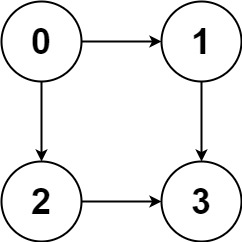``` Input: graph = [[1,2],,,[]] Output: [[0,1,3],[0,2,3]] Explanation: There are two paths: 0 -> 1 -> 3 and 0 -> 2 -> 3. ```

Example 2: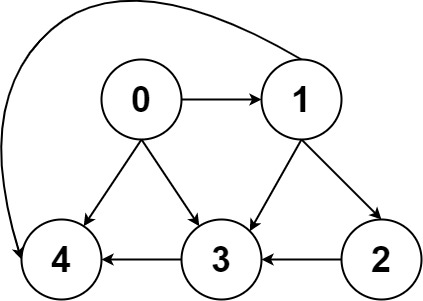``` Input: graph = [[4,3,1],[3,2,4],,,[]] Output: [[0,4],[0,3,4],[0,1,3,4],[0,1,2,3,4],[0,1,4]] ```

Example 3:

``` Input: graph = [,[]] Output: [[0,1]] ```

Example 4:

``` Input: graph = [[1,2,3],,,[]] Output: [[0,1,2,3],[0,2,3],[0,3]] ```

Example 5:

``` Input: graph = [[1,3],,,[]] Output: [[0,1,2,3],[0,3]] ```

2. All Paths Source Target – Solution

The following is a solution which demonstrates how to find all paths from source to target in a graph.

This solution uses Breadth First Search and backtracking when looking for paths.

``` 2. All Paths Source Target - Solution C# // ============================================================================ // Author: Kenneth Perkins // Date: Nov 28, 2021 // Taken From: http://programmingnotes.org/ // File: Solution.cs // Description: Demonstrates how to find all paths in a graph // ============================================================================ public class Solution { public IList<IList<int>> AllPathsSourceTarget(int[][] graph) { var result = new List<IList<int>>(); var queue = new Queue<List<int>>(); queue.Enqueue(new List<int>{0}); while (queue.Count > 0) { var currentPath = queue.Dequeue(); var currentNode = currentPath[currentPath.Count - 1]; if (currentNode == graph.Length - 1) { result.Add(currentPath); } else { foreach (var child in graph[currentNode]) { currentPath.Add(child); queue.Enqueue(new List<int>(currentPath)); currentPath.RemoveAt(currentPath.Count - 1); } } } return result; } }// http://programmingnotes.org/ 12345678910111213141516171819202122232425262728293031 // ============================================================================//    Author: Kenneth Perkins//    Date:   Nov 28, 2021//    Taken From: http://programmingnotes.org///    File:  Solution.cs//    Description: Demonstrates how to find all paths in a graph// ============================================================================public class Solution {    public IList<IList<int>> AllPathsSourceTarget(int[][] graph) {        var result = new List<IList<int>>();        var queue = new Queue<List<int>>();         queue.Enqueue(new List<int>{0});         while (queue.Count > 0) {            var currentPath = queue.Dequeue();            var currentNode = currentPath[currentPath.Count - 1];             if (currentNode == graph.Length - 1) {                result.Add(currentPath);            } else {                foreach (var child in graph[currentNode]) {                    currentPath.Add(child);                    queue.Enqueue(new List<int>(currentPath));                    currentPath.RemoveAt(currentPath.Count - 1);                }            }        }        return result;    }}// http://programmingnotes.org/ ```

QUICK NOTES:
The highlighted lines are sections of interest to look out for.

The code is heavily commented, so no further insight is necessary. If you have any questions, feel free to leave a comment below.

Once compiled, you should get this as your output for the example cases:

``` [[0,1,3],[0,2,3]] [[0,4],[0,3,4],[0,1,4],[0,1,3,4],[0,1,2,3,4]] [[0,1]] [[0,3],[0,2,3],[0,1,2,3]] [[0,3],[0,1,2,3]] ```

## C# || How To Capture All Surrounded Regions ‘X’ & ‘O’ In Board Matrix Using C#The following is a module with functions which demonstrates how to capture all surrounded regions ‘X’ and ‘O’ in a board matrix using C#.

1. Surrounded Regions – Problem Statement

Given an m x n matrix board containing ‘X’ and ‘O’, capture all regions that are 4-directionally surrounded by ‘X’.

A region is captured by flipping all ‘O’s into ‘X’s in that surrounded region.

Example 1: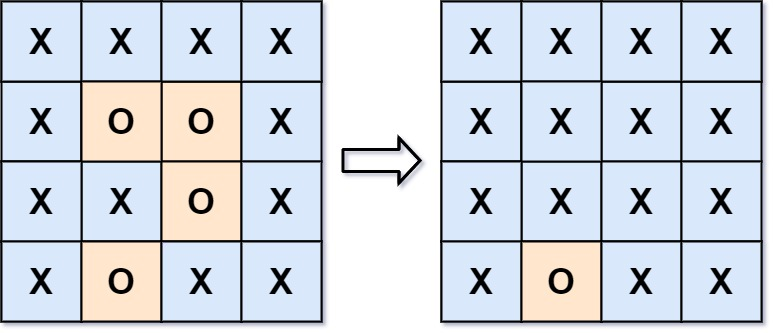``` Input: board = [["X","X","X","X"],["X","O","O","X"],["X","X","O","X"],["X","O","X","X"]] Output: [["X","X","X","X"],["X","X","X","X"],["X","X","X","X"],["X","O","X","X"]] Explanation: Surrounded regions should not be on the border, which means that any 'O' on the border of the board are not flipped to 'X'. Any 'O' that is not on the border and it is not connected to an 'O' on the border will be flipped to 'X'. Two cells are connected if they are adjacent cells connected horizontally or vertically. ```

Example 2:

``` Input: board = [["X"]] Output: [["X"]] ```

2. Surrounded Regions – Solution

The following is a solution which demonstrates how to capture all surrounded regions ‘X’ and ‘O’ in a board matrix.

This solution uses Breadth First Search when looking for surrounded regions.

A ‘O’ is surrounded if there is no path from it to the outer border of the matrix (i.e: row: index 0, column: index 0, row: index matrix.length-1, column: index matrix.length-1) when moving in a North, South, East, or West direction.

Basically:

``` A 'O' will not be flipped to 'X' if: It is on the border, OR It is connected to any other 'O' that cannot be flipped ```

In this solution we get all ‘O’ cells around the borders and keep track of all ‘O’ cells connected to the ones around the outer border.

We mark the border ‘O’ cells, and all the connected ‘O’ cells to the ones around the outer border as invalid.

In the end, we mark all valid ‘O’ cells as ‘X’.

``` 2. Surrounded Regions - Solution C# // ============================================================================ // Author: Kenneth Perkins // Date: Nov 1, 2021 // Taken From: http://programmingnotes.org/ // File: Solution.cs // Description: Demonstrates how to flip surrounded regions // ============================================================================ public class Solution { public void Solve(char[][] board) { var queue = new Queue<KeyValuePair<int, int>>(); // Get coordinates of 'O' cells on the left and right border and add to queue for (int row = 0; row < board.Length; ++row) { // Check left border if (board[row] == 'O') { queue.Enqueue(new KeyValuePair<int, int>(row, 0)); } // Check right border if(board[row][board[row].Length - 1] == 'O') { queue.Enqueue(new KeyValuePair<int, int>(row, board[row].Length - 1)); } } // Get coordinates of 'O' cells on the top and bottom border and add to queue for (int col = 0; col < board.Length; ++col) { // Check top border if (board[col] == 'O') { queue.Enqueue(new KeyValuePair<int, int>(0, col)); } // Check bottom border if (board[board.Length - 1][col] == 'O') { queue.Enqueue(new KeyValuePair<int, int>(board.Length - 1, col)); } } // 2D bool array to keep track of 'O' cells connected to the ones on the outer border var visited = new bool[board.Length][]; for (int row = 0; row < board.Length; ++row) { visited[row] = new bool[board[row].Length]; } // Search directions var directions = new List<KeyValuePair<int, int>>() { // Right new KeyValuePair<int, int>(0, 1), // Left new KeyValuePair<int, int>(0, -1), // Top new KeyValuePair<int, int>(-1, 0), // Bottom new KeyValuePair<int, int>(1, 0), }; // Find all 'O' cells connected to the ones on the outer border while (queue.Count > 0) { var current = queue.Dequeue(); // Get current coordinates var currentRow = current.Key; var currentCol = current.Value; // Mark cell as visited, which means its connected to a border cell visited[currentRow][currentCol] = true; // Search right, left, top, bottom for connected 'O' cells foreach (var direction in directions) { var newRow = currentRow + direction.Key; var newCol = currentCol + direction.Value; // Add connected cells to the queue if (IsValidCell(board, newRow, newCol) && board[newRow][newCol] == 'O' && !visited[newRow][newCol]) { queue.Enqueue(new KeyValuePair<int, int>(newRow, newCol)); } } } // Mark the 'O' cells not connected to outer borders as X for (int row = 0; row < board.Length; ++row) { for (int col = 0; col < board[row].Length; ++col) { if (board[row][col] == 'O' && !visited[row][col]) { board[row][col] = 'X'; } } } } private bool IsValidCell(char[][] board, int row, int col) { return row >= 0 && row < board.Length && col >= 0 && col < board[row].Length; } }// http://programmingnotes.org/ 123456789101112131415161718192021222324252627282930313233343536373839404142434445464748495051525354555657585960616263646566676869707172737475767778798081828384858687888990 // ============================================================================//    Author: Kenneth Perkins//    Date:   Nov 1, 2021//    Taken From: http://programmingnotes.org///    File:  Solution.cs//    Description: Demonstrates how to flip surrounded regions// ============================================================================public class Solution {    public void Solve(char[][] board) {        var queue = new Queue<KeyValuePair<int, int>>();         // Get coordinates of 'O' cells on the left and right border and add to queue        for (int row = 0; row < board.Length; ++row) {            // Check left border            if (board[row] == 'O') {                queue.Enqueue(new KeyValuePair<int, int>(row, 0));            }            // Check right border            if(board[row][board[row].Length - 1] == 'O') {                queue.Enqueue(new KeyValuePair<int, int>(row, board[row].Length - 1));            }        }         // Get coordinates of 'O' cells on the top and bottom border and add to queue        for (int col = 0; col < board.Length; ++col) {            // Check top border            if (board[col] == 'O') {                queue.Enqueue(new KeyValuePair<int, int>(0, col));            }            // Check bottom border            if (board[board.Length - 1][col] == 'O') {                queue.Enqueue(new KeyValuePair<int, int>(board.Length - 1, col));            }        }         // 2D bool array to keep track of 'O' cells connected to the ones on the outer border        var visited = new bool[board.Length][];        for (int row = 0; row < board.Length; ++row) {            visited[row] = new bool[board[row].Length];        }         // Search directions        var directions = new List<KeyValuePair<int, int>>() {            // Right            new KeyValuePair<int, int>(0, 1),            // Left            new KeyValuePair<int, int>(0, -1),            // Top            new KeyValuePair<int, int>(-1, 0),            // Bottom            new KeyValuePair<int, int>(1, 0),        };         // Find all 'O' cells connected to the ones on the outer border        while (queue.Count > 0) {            var current = queue.Dequeue();             // Get current coordinates            var currentRow = current.Key;            var currentCol = current.Value;             // Mark cell as visited, which means its connected to a border cell            visited[currentRow][currentCol] = true;             // Search right, left, top, bottom for connected 'O' cells            foreach (var direction in directions) {                var newRow = currentRow + direction.Key;                var newCol = currentCol + direction.Value;                 // Add connected cells to the queue                if (IsValidCell(board, newRow, newCol) && board[newRow][newCol] == 'O' && !visited[newRow][newCol]) {                    queue.Enqueue(new KeyValuePair<int, int>(newRow, newCol));                }            }        }         // Mark the 'O' cells not connected to outer borders as X        for (int row = 0; row < board.Length; ++row) {            for (int col = 0; col < board[row].Length; ++col) {                if (board[row][col] == 'O' && !visited[row][col]) {                    board[row][col] = 'X';                }            }        }    }     private bool IsValidCell(char[][] board, int row, int col) {        return row >= 0 && row < board.Length && col >= 0 && col < board[row].Length;    }}// http://programmingnotes.org/ ```

QUICK NOTES:
The highlighted lines are sections of interest to look out for.

The code is heavily commented, so no further insight is necessary. If you have any questions, feel free to leave a comment below.

Once compiled, you should get this as your output for the example cases:

``` [["X","X","X","X"],["X","X","X","X"],["X","X","X","X"],["X","O","X","X"]] [["X"]] ```